# ISEE Lower Level Quantitative : How to find a parallelogram on a coordinate plane

## Example Questions

### Example Question #1 : Coordinate Geometry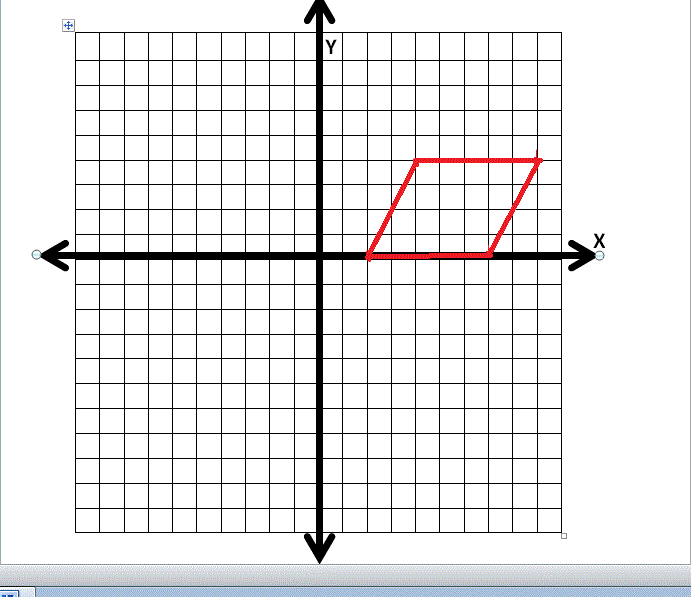The parallelogram shown above has a height ofand a base of length. Find the area of the parallelogram.square unitssquare unitssquare unitssquare unitssquare units

Explanation:

To find the area of the parallelogram apply the formula: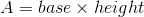Since, the paralleogram has a base ofand a height ofthe solution is: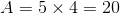### Example Question #2 : Coordinate GeometryThe parallelogram shown above has a height ofand a base of length. Find the perimeter of the parallelogram.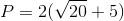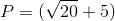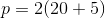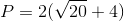Explanation:

In order to find the correct perimeter of the parallelogram apply the formula: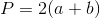, where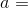the length of one of the diagonal sides and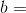the length of the base.

In order to find the length of side, apply the formula: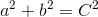. By drawing an altitude from point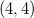to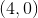, a right triangle is formed with a base that has a length ofand a height of.

Thus, the solution is: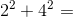length of side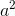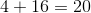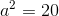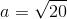Therefore,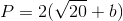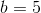### Example Question #3 : Coordinate Geometry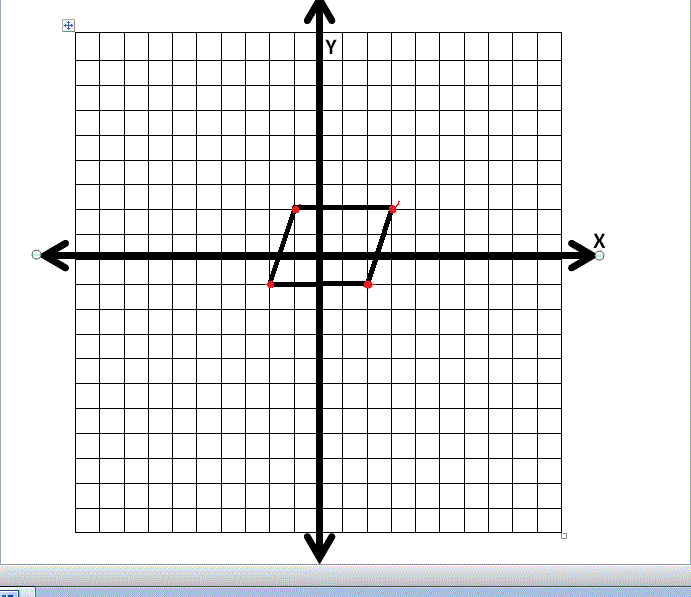Identify the coordinate points for the parallelogram that is shown above.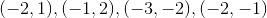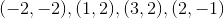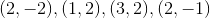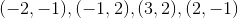Explanation:

In order to identify the coordinate points for this parallelogram, notice that there must be two different pairs of coordinates with the samevalues.

Thus, the parallelogram has coordinate points:### Example Question #4 : Coordinate GeometryWhat is the area of the parallelogram shown above?square unitssquare unitssquare unitssquare unitssquare units

Explanation:

To find the area of the parallelogram that is shown, apply the formula:Since the parallelogram has a base ofand a height ofthe solution is: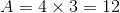### Example Question #5 : Coordinate GeometryGiven that the above parallelogram has base sides with a length ofand diagonal sides with a length of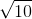what is the perimeter of the parallelogram?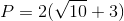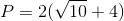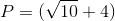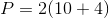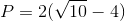Explanation:

In order to find the perimeter of the parallelogram apply the formula:, wherethe length of one diagonal side andthe length of one base.

In this problem,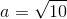and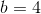.
Thus, the correct answer is:### Example Question #6 : Coordinate GeometryIdentify the coordinate points for the parallelogram shown above.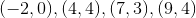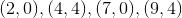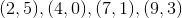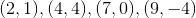In order to identify the coordinate points for this parallelogram, notice that there must be two different pairs of coordinates with the samevalues.